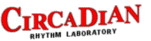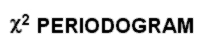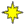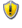To enjoy this web site, please instruct your browser to accept scripts.
 This section contains an on-line version of the chi-square periodogram test for the computation of circadian period.
 The program requires a VBScript-enabled browser. Your browser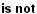VBScript enabled.
Instructions
 This program will compute the circadian period of a time series and will determine the statistical significance of the computed period. The program is an implementation of the chi-square periodogram procedure (see references on the right panel). Equally-spaced data sets (with one measurement per line) are expected. The time of day when the data set starts is irrelevant. The resolution of the computed period will depend on the resolution (bin size) of your data. For a resolution of 0.1 hour, you need a bin size of 6 minutes. Regardless of the resolution of the computations, the test of significance (used to determine whether the computed period is statistically significant) assumes that you have at least 10 days of data. With fewer days, the computed value will still be accurate, but the significance test will be less sensitive.

References
 1) Enright, J. T. (1965). The search for rhythmicity in biological time-series. Journal of Theoretical Biology 8: 426-468. 2) Sokolove, P. G. and Bushell, W. N. (1978). The chi square periodogram: its utility for analysis of circadian rhythms. Journal of Theoretical Biology 72: 131-160. 3) Refinetti, R. (1993). Comparison of six methods for the determination of the period of circadian rhythms. Physiology and Behavior 54: 869-875. 4) Refinetti, R. (2004). Non-stationary time series and the robustness of circadian rhythms. Journal of Theoretical Biology 227: 571-581.
Program
 1) Type or paste the data here: 2) Enter the temporal resolution of the data: Bin size (min): 3)   4) See the results:

Note
 The test of significance assumes that you have at least 10 days of data. With fewer days, the computed value will still be accurate, but the significance test will be less sensitive. The probability indicated here incorporates correction for multiple testing (all periods between 19 and 27 hours). The need for this correction is arguable. If the test fails to identify significant rhythmicity, you may try a more lenient level of significance. The critical value of Qp to be exceeded at a given significance level is the value of the chi-square statistic for (P − 1) degrees of freedom (where P is the period in number of bins).
 To find out the probability associated with a given Qp value with no correction for multiple periods, you may use this calculator (approximate values): Qp value: Period (in bins):